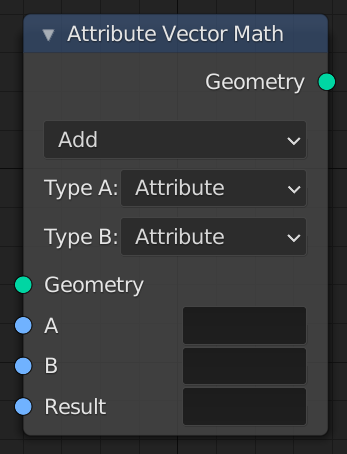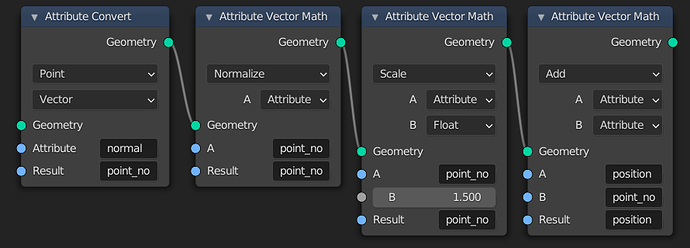# Attribute Vector Math¶

Modify an attribute with a math operation.The Attribute Vector Math Node.

## Inputs¶

Geometry

Standard geometry input.

A, B, C

The inputs to the math operations. Depending on the operation one, two, or all three of the inputs will be used. The attribute types are all vectors of three values, except for the Scale operation, where the second input uses a float type.

Result

The name of the attribute where the computed result it stored. A new attribute with that name is added if it does not exist yet. If it does exist, the values of the existing attribute are overridden.

## Properties¶

Operation

The math function to perform.

The sum of A and B.

Subtract

The difference between A and B.

Multiply

The entrywise product of A and B. $$(A.x * B.x, A.y * B.y, A.z * B.z)$$

Divide

The entrywise division of A by B. Division by zero results in zero. $$(A.x / B.x, A.y / B.y, A.z / B.z)$$

Cross Product

The cross product of A and B.

Project

The projection of A onto B.

Reflect

The reflection of A around the normal B. B need not be normalized.

Refract

For a given incident vector A, surface normal B and ratio of indices of refraction (IOR) refract outputs the refraction vector R.

Faceforward

Orients a vector A to point away from a surface B as defined by its normal C. Computes $$(dot(B, C) < 0) ? A : -A$$.

Dot Product

The dot product of A and B.

Distance

The distance between A and B.

Length

The length of A.

Scale

The result of multiplying A by the scalar input Scale.

Normalize

The result of normalizing A.

Wrap

Wrap.

Snap

The result of rounding A to the largest integer multiple of B less than or equal A.

Floor

The entrywise floor of A.

Ceil

The entrywise ceiling of A.

Modulo

The entrywise modulo of A by B.

Fraction

The fractional part of A.

Absolute

The entrywise absolute value of A.

Minimum

The entrywise minimum from A and B.

Maximum

The entrywise maximum from A and B.

Sine

The entrywise Sine of A.

Cosine

The entrywise Cosine of A.

Tangent

The entrywise Tangent of A.

Note

Attributes are converted implicitly to the input data type.

A, B, C
Attribute

A text field to input an attribute name.

Vector

The input is a vector of three float numbers.

## Output¶

Geometry

Standard geometry output.

## Example¶

Here are nodes to move points along the normals of a mesh or points from the Point Distribute node. First the normal attribute is moved to the Point domain. Then it is normalized, the length is changed, and it is added to the position. The Factor input could instead be an attribute to vary the displacement per point.